# Surface Area and Volume Surface Area of Prisms

• Slides: 46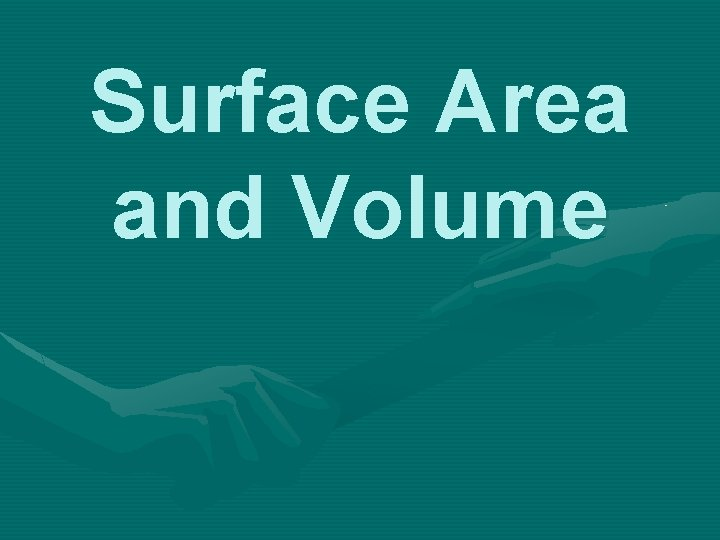Surface Area and VolumeSurface Area of Prisms Surface Area = The total area of the surface of a three-dimensional object (Or think of it as the amount of paper you’ll need to wrap the shape. ) Prism = A solid object that has two identical ends and all flat sides. We will start with 2 prisms – a rectangular prism and a triangular prism.Rectangular Prism Triangular PrismSurface Area (SA) of a Rectangular Prism Like dice, there are six sides (or 3 pairs of sides)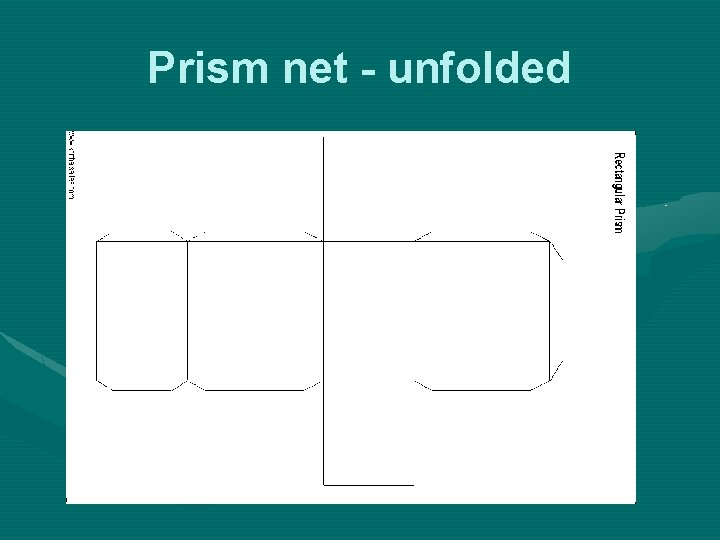Prism net - unfolded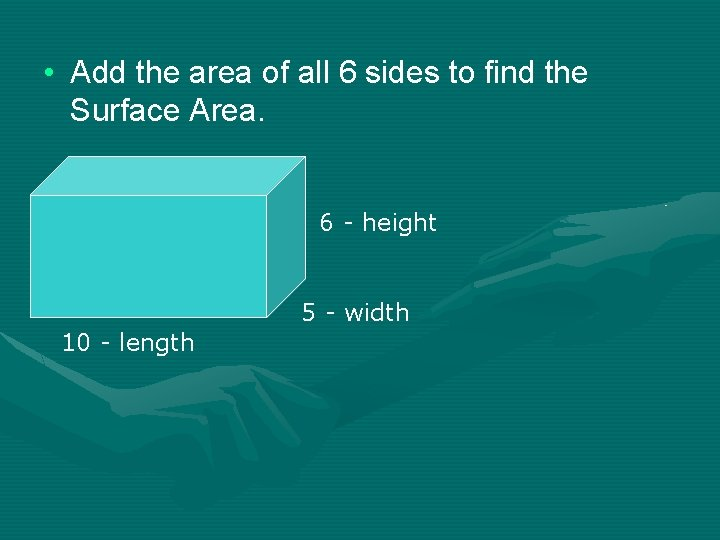• Add the area of all 6 sides to find the Surface Area. 6 - height 5 - width 10 - length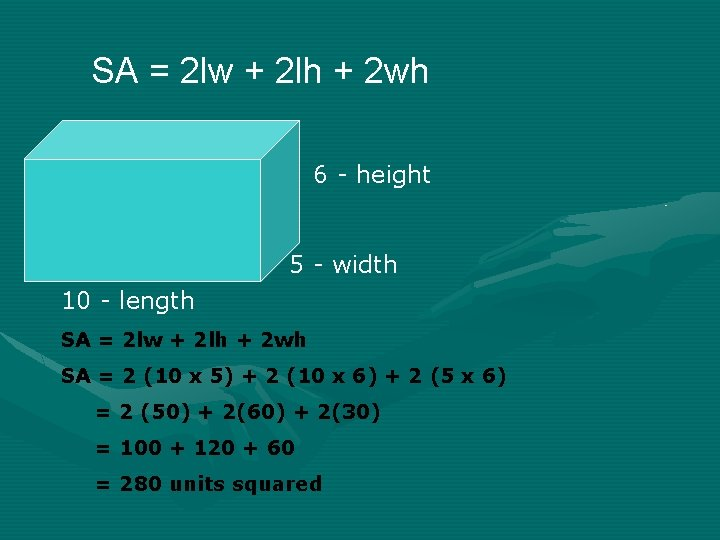SA = 2 lw + 2 lh + 2 wh 6 - height 5 - width 10 - length SA = 2 lw + 2 lh + 2 wh SA = 2 (10 x 5) + 2 (10 x 6) + 2 (5 x 6) = 2 (50) + 2(60) + 2(30) = 100 + 120 + 60 = 280 units squaredPractice 12 ft 10 ft 22 ft SA = 2 lw + 2 lh + 2 wh = 2(22 x 10) + 2(22 x 12) + 2(10 x 12) = 2(220) + 2(264) + 2(120) = 440 + 528 + 240 = 1208 ft squaredSurface Area of a Triangular Prism • 2 bases (triangular) • 3 sides (rectangular)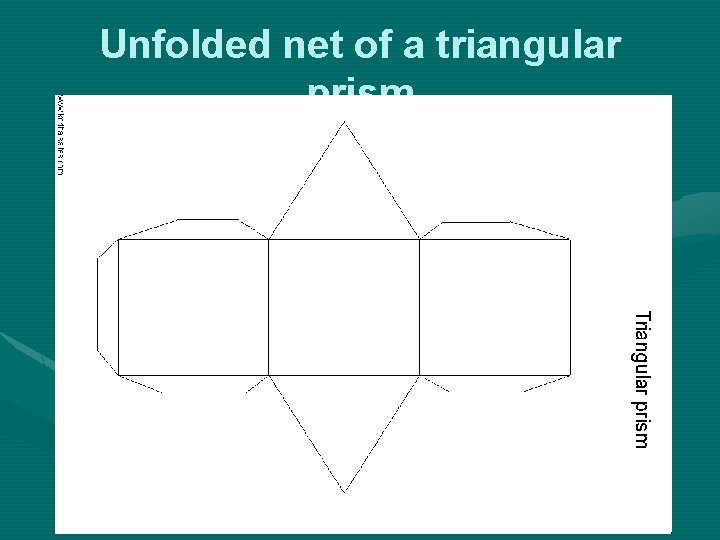Unfolded net of a triangular prism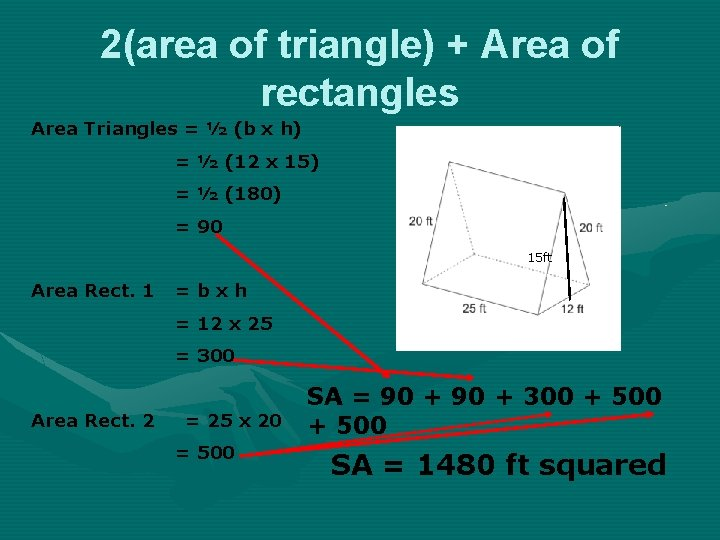2(area of triangle) + Area of rectangles Area Triangles = ½ (b x h) = ½ (12 x 15) = ½ (180) = 90 15 ft Area Rect. 1 =bxh = 12 x 25 = 300 Area Rect. 2 = 25 x 20 = 500 SA = 90 + 300 + 500 SA = 1480 ft squared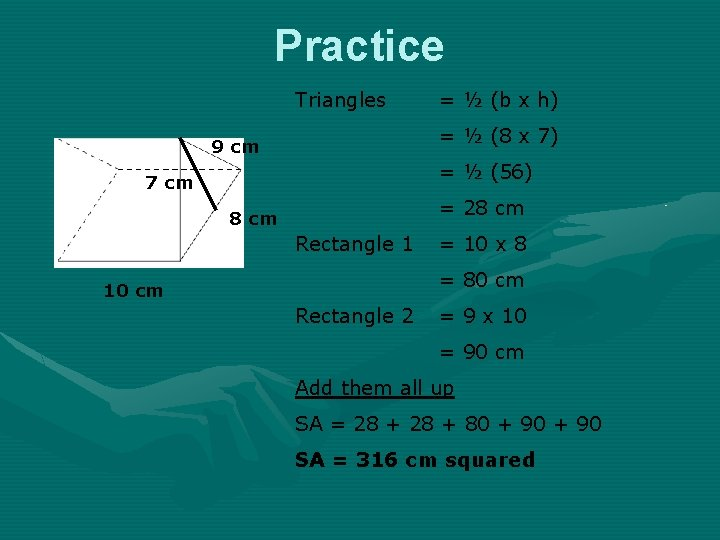Practice Triangles = ½ (b x h) = ½ (8 x 7) 9 cm = ½ (56) 7 cm = 28 cm Rectangle 1 = 10 x 8 = 80 cm 10 cm Rectangle 2 = 9 x 10 = 90 cm Add them all up SA = 28 + 80 + 90 SA = 316 cm squared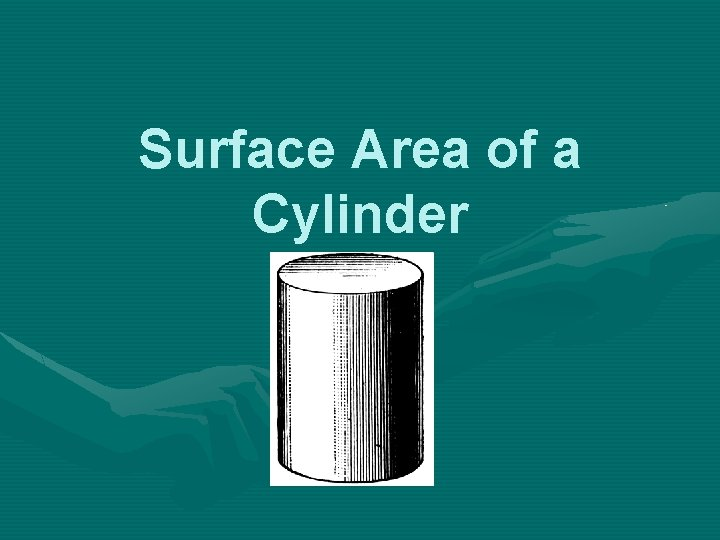Surface Area of a Cylinder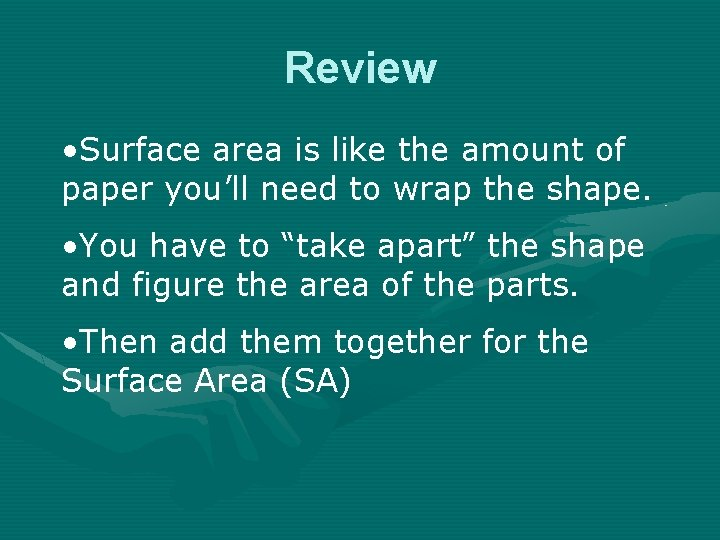Review • Surface area is like the amount of paper you’ll need to wrap the shape. • You have to “take apart” the shape and figure the area of the parts. • Then add them together for the Surface Area (SA)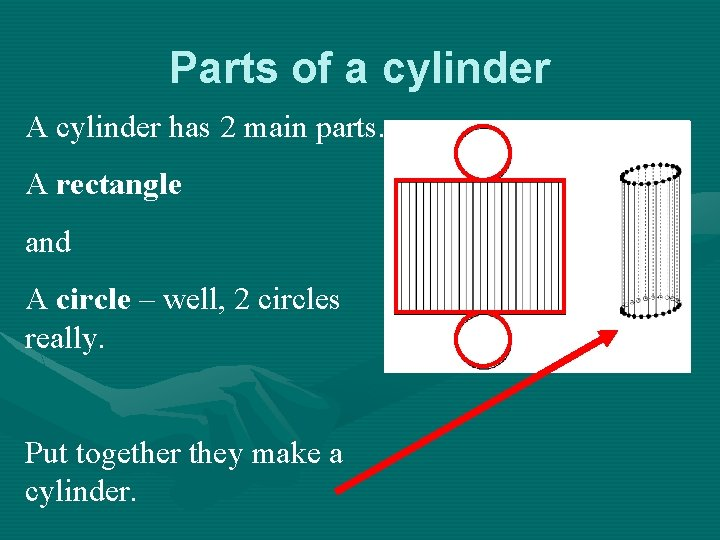Parts of a cylinder A cylinder has 2 main parts. A rectangle and A circle – well, 2 circles really. Put together they make a cylinder.The Soup Can Think of the Cylinder as a soup can. You have the top and bottom lid (circles) and you have the label (a rectangle – wrapped around the can). The lids and the label are related. The circumference of the lid is the same as the length of the label.Area of the Circles Formula for Area of Circle A= r 2 = 3. 14 x 32 = 3. 14 x 9 = 28. 26 But there are 2 of them so 28. 26 x 2 = 56. 52 units squared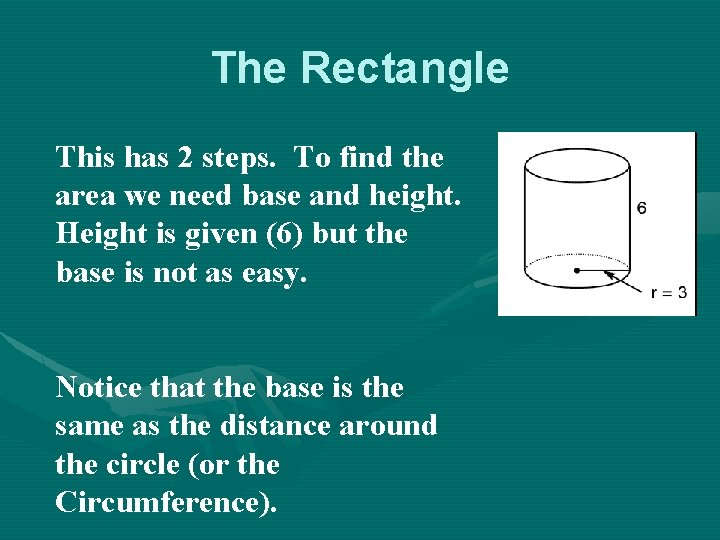The Rectangle This has 2 steps. To find the area we need base and height. Height is given (6) but the base is not as easy. Notice that the base is the same as the distance around the circle (or the Circumference).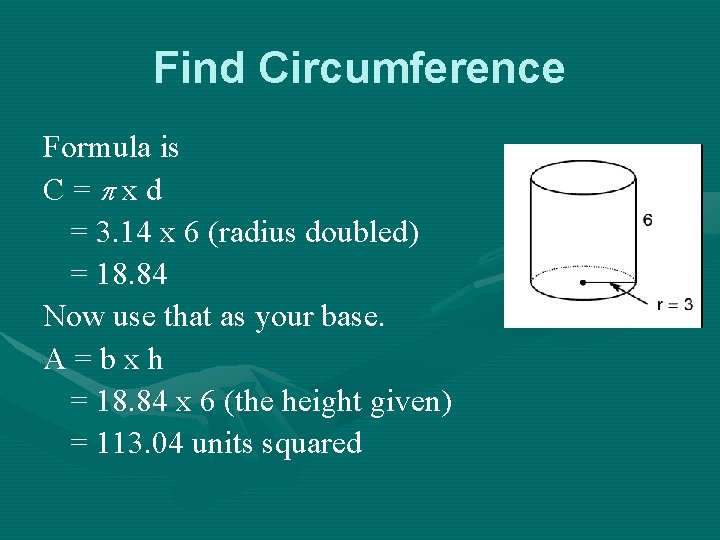Find Circumference Formula is C= xd = 3. 14 x 6 (radius doubled) = 18. 84 Now use that as your base. A=bxh = 18. 84 x 6 (the height given) = 113. 04 units squared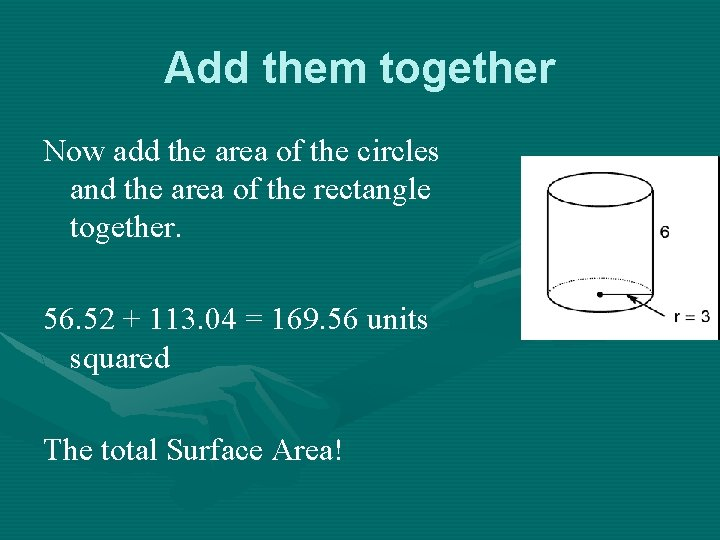Add them together Now add the area of the circles and the area of the rectangle together. 56. 52 + 113. 04 = 169. 56 units squared The total Surface Area!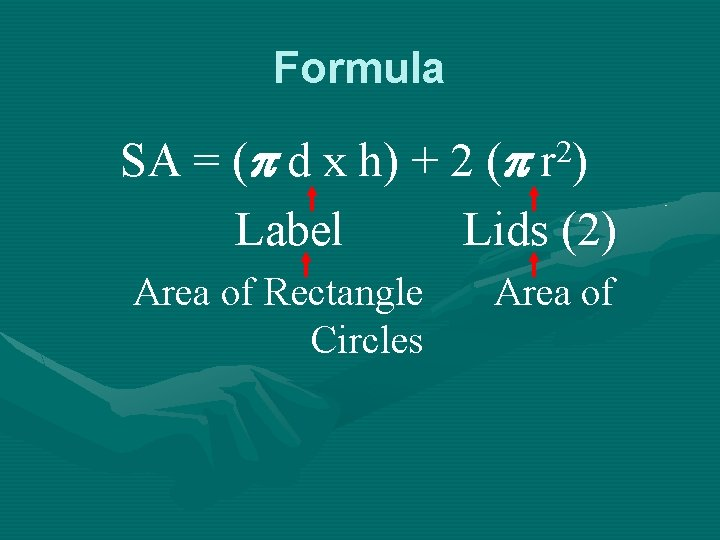Formula SA = ( d x h) + 2 ( r 2) Label Lids (2) Area of Rectangle Circles Area of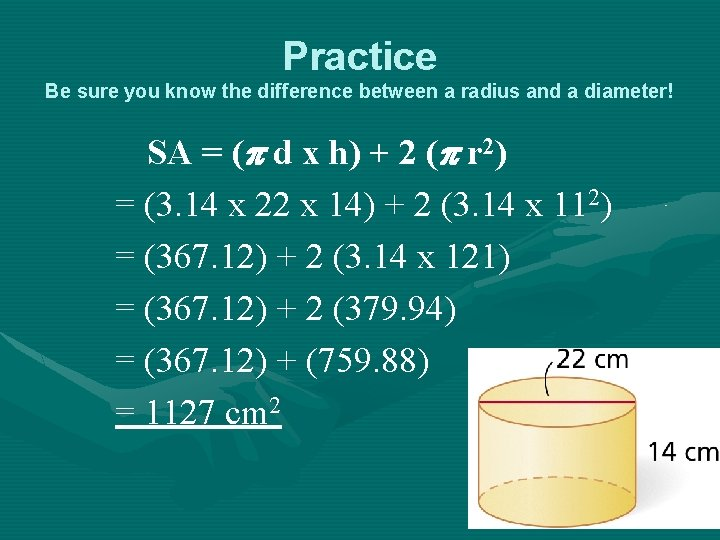Practice Be sure you know the difference between a radius and a diameter! SA = ( d x h) + 2 ( r 2) = (3. 14 x 22 x 14) + 2 (3. 14 x 112) = (367. 12) + 2 (3. 14 x 121) = (367. 12) + 2 (379. 94) = (367. 12) + (759. 88) = 1127 cm 2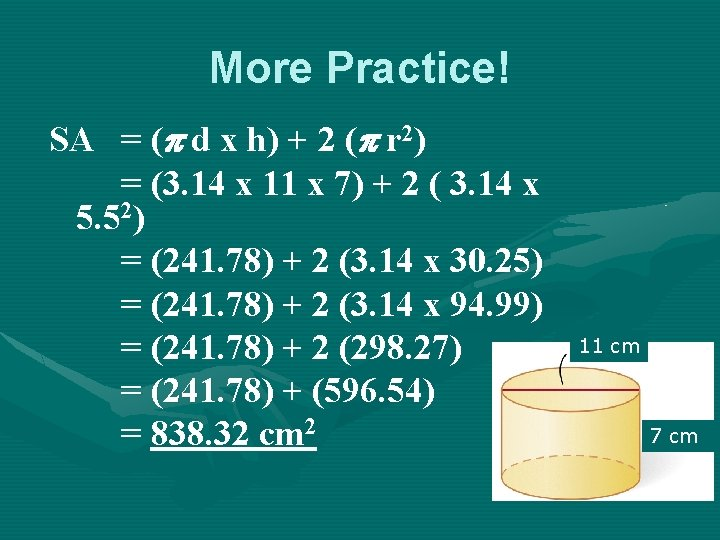More Practice! SA = ( d x h) + 2 ( r 2) = (3. 14 x 11 x 7) + 2 ( 3. 14 x 5. 52) = (241. 78) + 2 (3. 14 x 30. 25) = (241. 78) + 2 (3. 14 x 94. 99) = (241. 78) + 2 (298. 27) = (241. 78) + (596. 54) = 838. 32 cm 2 11 cm 7 cm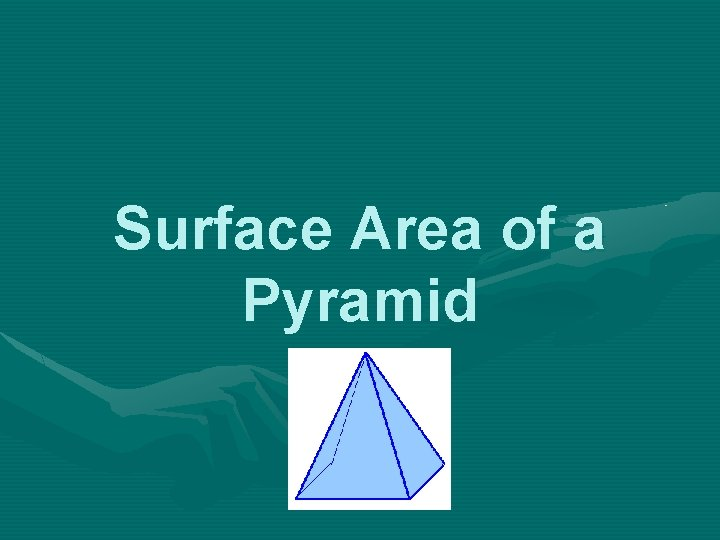Surface Area of a Pyramid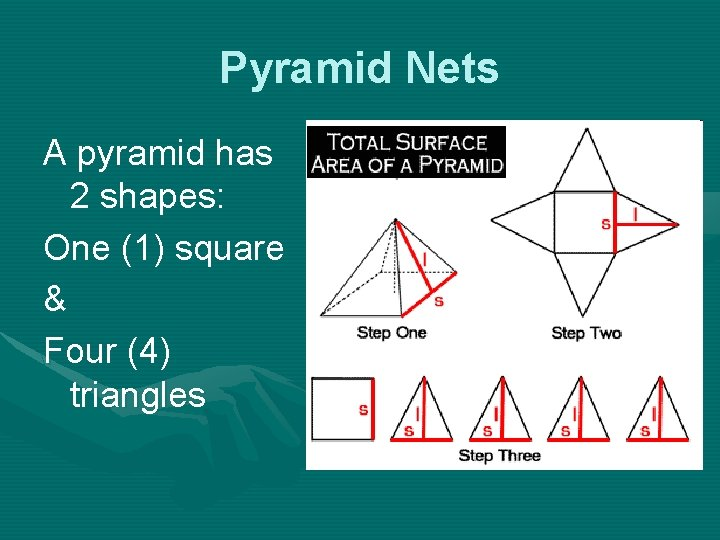Pyramid Nets A pyramid has 2 shapes: One (1) square & Four (4) triangles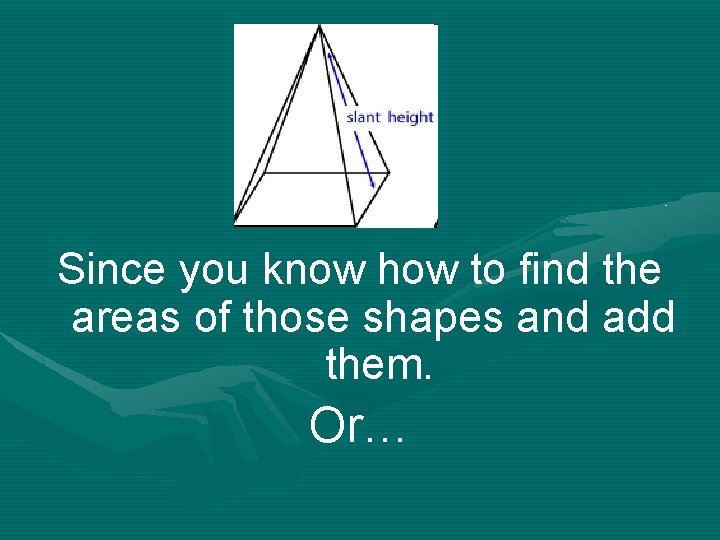Since you know how to find the areas of those shapes and add them. Or…you can use a formula… SA = ½ lp + B Where l is the Slant Height and p is the perimeter and B is the area of the Base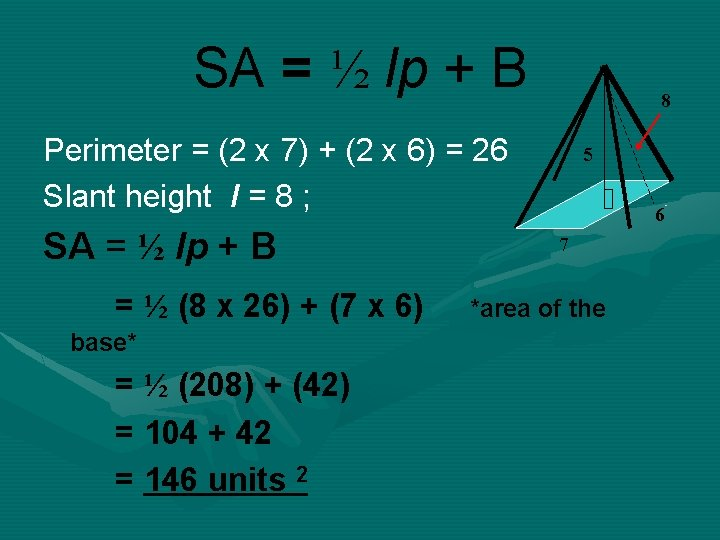SA = ½ lp + B 8 Perimeter = (2 x 7) + (2 x 6) = 26 Slant height l = 8 ; SA = ½ lp + B = ½ (8 x 26) + (7 x 6) base* = ½ (208) + (42) = 104 + 42 = 146 units 2 5 6 7 *area of the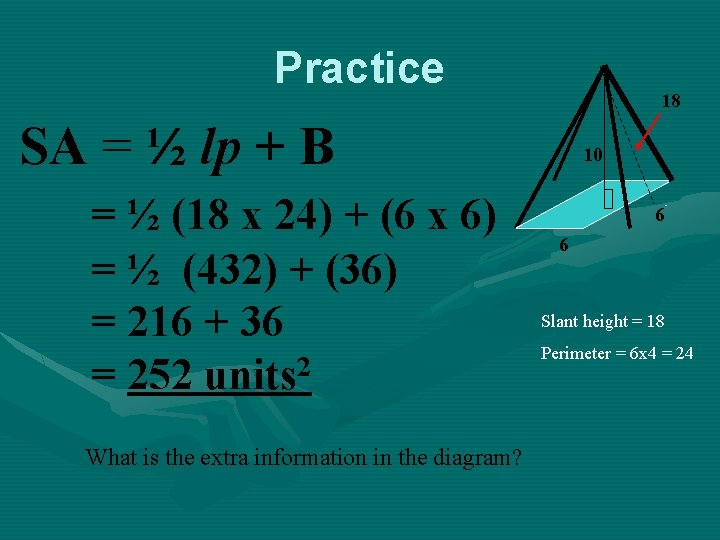Practice 18 SA = ½ lp + B = ½ (18 x 24) + (6 x 6) = ½ (432) + (36) = 216 + 36 = 252 units 2 What is the extra information in the diagram? 10 6 6 Slant height = 18 Perimeter = 6 x 4 = 24Volume of Prisms and CylindersVolume • The number of cubic units needed to fill the shape. Find the volume of this prism by counting how many cubes tall, long, and wide the prism is and then multiplying. • There are 24 cubes in the prism, so the volume is 24 cubic units. 2 x 3 x 4 = 24 2 – height 3 – width 4 – lengthFormula for Prisms VOLUME OF A PRISM The volume V of a prism is the area of its base B times its height h. V = Bh Note – the capital letter stands for the AREA of the BASE not the linear measurement.Try It 3 ft - height 4 ft width 8 ft - length V = Bh Find area of the base = (8 x 4) x 3 = (32) x 3 Multiply it by the height = 96 ft 3Practice V 12 cm 10 cm 22 cm = Bh = (22 x 10) x 12 = (220) x 12 = 2640 cm 3Cylinders VOLUME OF A CYLINDER The volume V of a cylinder is the area of its base, r 2, times its height h. V = r 2 h Notice that r 2 is the formula for area of a circle.Try It V = r 2 h The radius of the cylinder is 5 m, and the height is 4. 2 m V = 3. 14 · 52 · 4. 2 Substitute the values you V = 329. 7 know.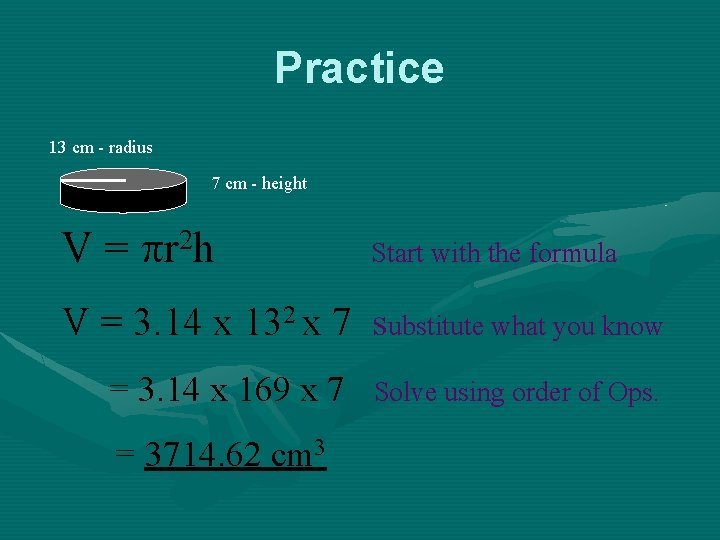Practice 13 cm - radius 7 cm - height V = r 2 h Start with the formula V = 3. 14 x 132 x 7 substitute what you know = 3. 14 x 169 x 7 = 3714. 62 cm 3 Solve using order of Ops.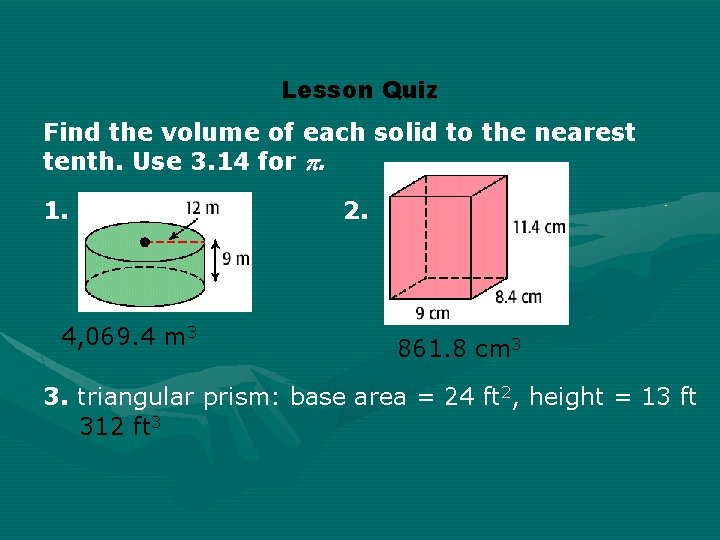Lesson Quiz Find the volume of each solid to the nearest tenth. Use 3. 14 for . 1. 4, 069. 4 m 3 2. 861. 8 cm 3 3. triangular prism: base area = 24 ft 2, height = 13 ft 312 ft 3Volume of PyramidsRemember that Volume of a Prism is B x h where b is the area of the base. You can see that Volume of a pyramid will be less than that of a prism. How much less? Any guesses?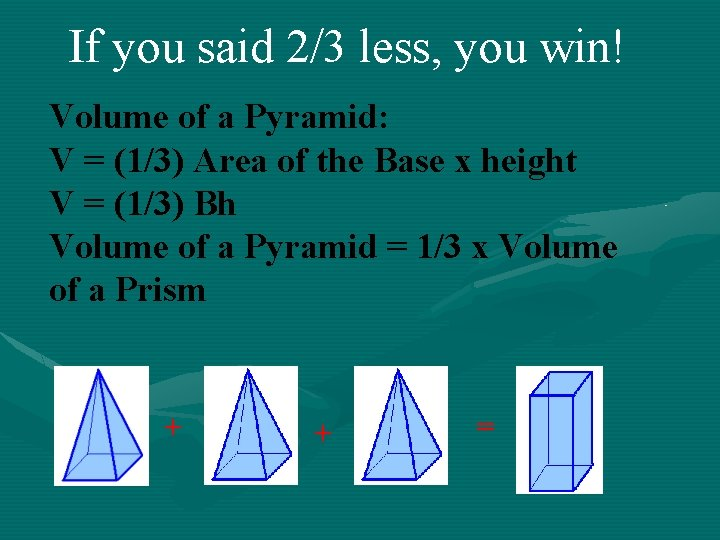If you said 2/3 less, you win! Volume of a Pyramid: V = (1/3) Area of the Base x height V = (1/3) Bh Volume of a Pyramid = 1/3 x Volume of a Prism + + =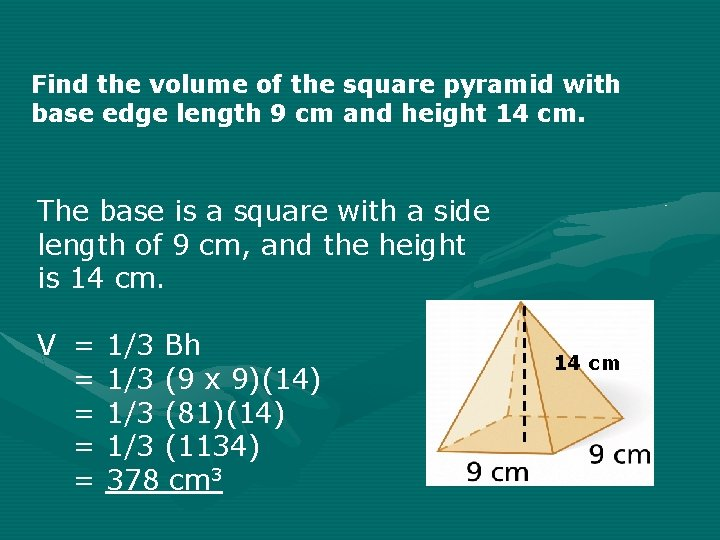Find the volume of the square pyramid with base edge length 9 cm and height 14 cm. The base is a square with a side length of 9 cm, and the height is 14 cm. V = = = 1/3 Bh 1/3 (9 x 9)(14) 1/3 (81)(14) 1/3 (1134) 378 cm 3 14 cmPractice V = 1/3 Bh = 1/3 (5 x 5) (10) = 1/3 (25)(10) = 1/3 250 = 83. 33 units 3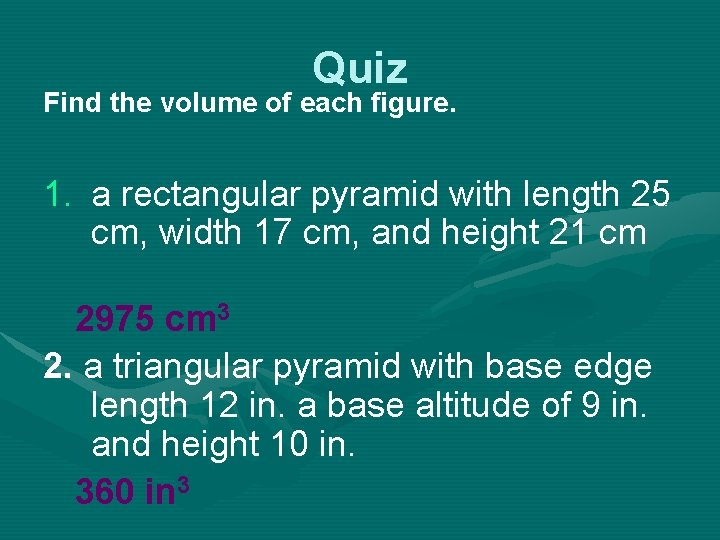Quiz Find the volume of each figure. 1. a rectangular pyramid with length 25 cm, width 17 cm, and height 21 cm 2975 cm 3 2. a triangular pyramid with base edge length 12 in. a base altitude of 9 in. and height 10 in. 360 in 3End